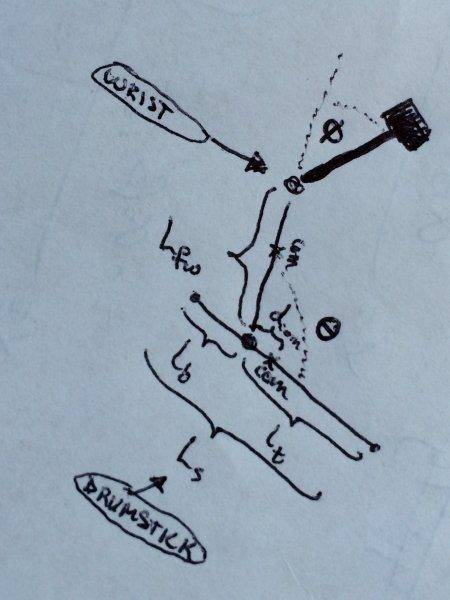# Kinetic Energy of Double Compound Pendulum and Parallel Axis

Kinetic Energy of Double Compound Pendulum and Parallel Axis Theorem

Hello, there.

I have a project I'm working on where I need to be able to calculate the kinetic energy of what basically amounts a double compound pendulum. However, the pivot point of the second pendulum is not at the center of mass (ergo the necessity of using the parallel axis theorem). Furthermore, because of the way the system is set up, the angles θ1 and θ2 are more like deviations from 'rest' positions that are not parallel with the force of gravity. I tried reading up on the equation for a double compound pendulum and the parallel axis theorem online, but nowhere, I believe, have I found an article combining the two concepts in the way I have in mind, not even counting the angle condition I was wanting to set up. Would anyone be able to give me some pointers? I'm not afraid to do a little reading, if need be.

(Note: Please know that I have a good familiarity with algebra. Calculus, not so much but I know just enough of that to maybe get an idea of what's going on.)

OldEngr63
Gold Member
Do you have a drawing of your system? Please post a figure.It's just a system of someone holding a drumstick, where only their fingers and wrist are seen as being able to pivot. Θ should probably instead be the acute angle on the other side, Θ and Φ are both the rest angles of their particular joint no greater than 90°/0.5π or less than 0 each, and only... increase from those values, if pivoting using that part of the hand, then return either Θ or Φ, respectively.

While I would LOVE to be able to extend this out to calculate what it would be like if the elbow was also pivoting (or just the elbow and fingers, with the wrist 'locked' either at rest or another angle), I've seen the equation for motion of a double compound pendulum, and I get the feeling that the complexity might increase exponentially, and then much, MUCH more if, say, the fingers and wrist were locked but the elbow and SHOULDER were to pivot instead (assuming, of course, that they were to all rotate in the same plane)...